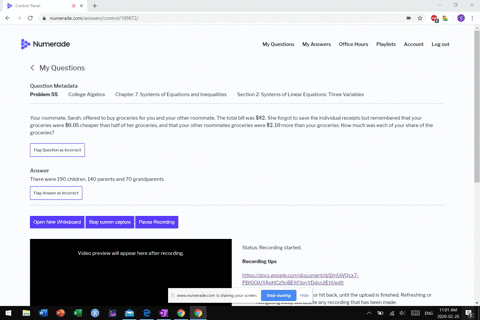Enroll in one of our FREE online STEM bootcamps. Join today and start acing your classes!View Bootcamps02:59
MB
Problem 54

# An animal shelter has a total of 350 animals comprised of cats, dogs, and rabbits. If the number of rabbits is 5 less than one-half the number of cats, and there are 20 more cats than dogs, how many of each animal are at the shelter?

## Discussion

You must be signed in to discuss.

## Video Transcript

All right. So Question 54. Got a word problem again with three variables. Three variables are three different types of animals. So we know that a certain animal shelter there are 350 animals. The rabbits that are in the shelter or, um, are five less than half the number of cats and their 20 more cats than dogs s. So we have to set up our variables. Um, I decided arbitrarily to make sure that to have x equal the rabbits. Why cats and people. The number dogs. All right, so our first equation is gonna be that x plus. Why? What Z equals 3 50 Okay, now the rabbits are five less than half the cats. So the rabbits you won't. Five less than five. Less than half that. Yes. And then finally, there's 20 more tests and dogs case. That means that, um, dogs plus 20 people's cats. So come, basically what we want a deal here is to start substitute into the top equation. So what we already have here is this in or right here? And so it's sending X plus y. It's gonna be 1/2. Why Minors fire men. Plus why and then we want to solve this one for Z so we can service to the Y value for that Z is why minus 20. So for us. Why? Minus 20? He was 35 our 350 50. All right, Um, now, once we have that, uh, let's go here. Combined terms. Eso We've got 122 and 1/2. Five over two. Why? Um You see, we've got minus 25 on this side. So let's had 25 this side that he's 3 75 Multiply both sides reciprocal here. And why equals 375 sounds too, We're told. Love. Oh, turned off 3 75 away. 6 375 3 75 by five times two. Here's 1 50 Okay, so there are 100 and 50 cats. You see, there's that's 20 more and the dogs. Okay, so why minus 20? So 130 you dogs and then half of, um, they have to have 23 fifties of this next class. One has to be seven. The second makes two 1 50 75 50. Just 200 left. Okay. All right. Sorry about that. Okay. Check